# How Subtraction Works

Page 1 of 3
This is the very beginning of understanding how subtraction works.

Let's look at this set: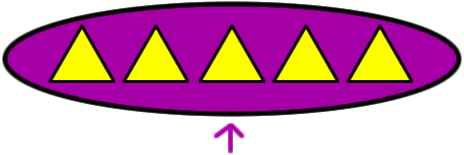Count how many triangles
are in this set...  There are 5!

Now, take two triangles away...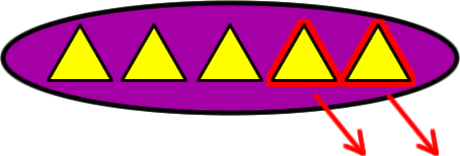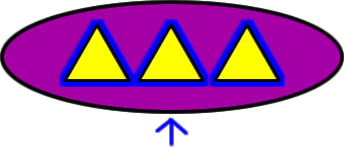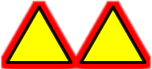Count how many triangles are left in the set...  There are 3 left!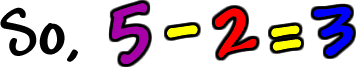```If you have troubles understanding subtractions,
check the best math homework help websites and one of them can assist you with this.```